# CBSE 9th Physics | Motion – Solved Problems

## Motion – Solved Problems

### Problem 1. A car covers the 1st half of the distance between two places at a speed of 40 km/hr and the 2nd half at 60 km/hr what is the average speed of the car ?

Solution.

Suppose the total distance covered is S. Then time taken to cover first’s distance with speed

40 km/hr.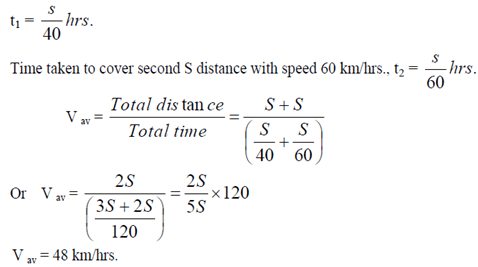### Problem 2. An object moving to the right has a decrease in velocity from 5.0 m/s to 1.0 m/s in 2.0 s. What is the average acceleration ? What does your result mean ?

Solution.

Given v0 = + 5.0 m/s, v = + 1.0 m/s, t = 2.0 s

According to the definition of average acceleration,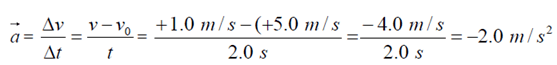The negative sign means the acceleration is opposite to velocity (deceleration). The result means that the object decreases its velocity by 2.0 m/s every s or 2.0 m/s2.

### Problem 3. The table below shows the distance in cm, travelled by the objects A, B and C during each second.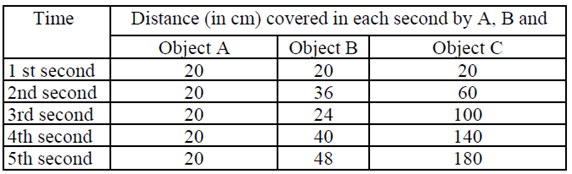#### (iii) Which object is moving with irregular acceleration ?

Solution

The object A is moving with constant speed. The reason is that it covers equal distance=20cm. in each second,

The object C is moving with a constant acceleration. The reason is that for the object C, the distance covered increases by the same amount in each second. It can further be verified by drawing graph between S (total distance covered) and t2 (square of time taken). The graph will be a straight line.

 t2 1 4 9 16 25 s 20 80 180 320 500

The object B is moving with irregular acceleration.

### Question 4. An automobile accelerates uniformly from rest to 25 m/s while traveling 100m. What is the acceleration of the automobile ?

Solution.

Given: v0 = 0 (rest), v = 25m/s, x = 100m. Find a.

Since v2 = v02 + 2ax,Since a is positive, it is in the direction of the velocity or motion.

### Problem 5. car is moving at a speed 50 km/h. Two seconds there after it is moving at 60 km/h. Calculate the acceleration of the car.

Solution.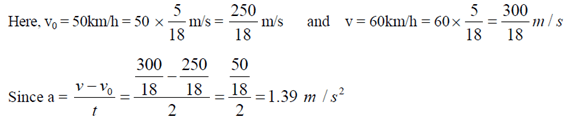### Problem 6. A bus moving with a velocity of 60 km/h is brought to rest in 20 seconds by applying brakes. Find its acceleration.

Solution.### Problem 7. A bullet moving with 10 m/s hits the wooden plank the bullet is stopped when it penetrates the plank 20 cm. deep, calculate retardation of the bullet.

Solution.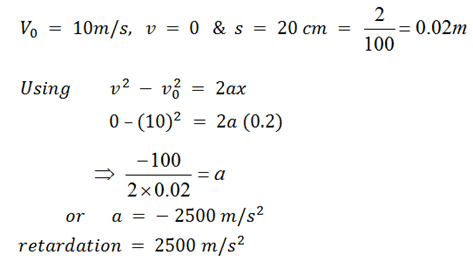### Problem 8. A body with an initial velocity of 18 km/hr accelerates uniformly at the rate of 9 cm s-2 over a distance of 200m. Calculate:

#### (ii) its final velocity in ms-1

Solution. (i)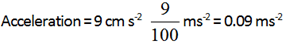Solution (ii)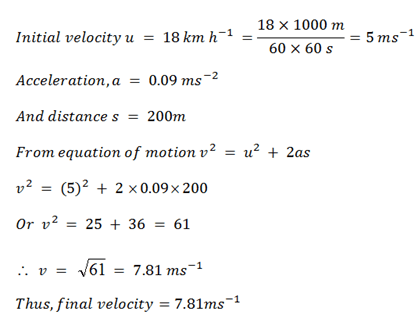### Problem 9. The graph represents the velocity of a particle as a function of time.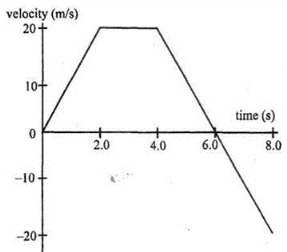#### (e) What is the displacement for the 8.0 s interval ?

Solution. (a)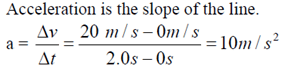Solution (b)

The slope of the line is zero and a = 0.

Solution (c)Solution (d)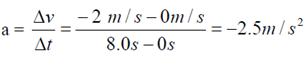Solution (e)This site uses Akismet to reduce spam. Learn how your comment data is processed.